# Teaching in USA

## TEACHING IN FIU

 The courses delivered during 1991-1998 (the number in brackets shows the average of student evaluations in the scale of five) MS level 1. Intermediate Analysis of     Mechanical Systems (3 semesters, 4.39) 2. Synthesis of Engineering     Mechanics (3 semesters, 4.49) 3 Principles of Composite Materials    (1 semester 4.38) 4. Classical Dynamics (1 semester, 4.68) Field Theory MS / PhD level 1. Advanced Analysis of Mechanical Systems     (3 semesters, 4.29) 2. Fracture Mechanics (3 semesters, 4.90) 3. Advanced Mechanical Vibrations     Analysis (3 semesters, 4.81 4. Fatigue and Failure Analysis     (2 semesters, 4.75) 5. Conduction Heat Transfer     (1 semester, 4.67) 6. Advanced Theory of Elasticity     (2 semesters, 4.71) PhD level 1. Boundary Value Problems of     Function Theory (1semester, 5.00) 2. Advanced Fracture Mechanics     (2 semesters, 4.9) 3. Boundary Problems in Engineering     (1 semester, 4.5) 4. Mechanics of Vortex and Separated Flows     (1 semester, 5.00) Undergraduate level 1. Mechanical Vibration Analysis     (3 semesters, 4.35) 2. Mechanics and Materials Science     (1 semester) The new courses offered by Dr.Cherepanov and approved by the Curriculum Committee Undergraduate level Gas Dynamics     MS level Field Theory     MS / PhD level 1. Advanced Mechanics of     Composite Materials 2. Mechanics of Flow in     Porous Materials   PhD level 1. Classical Topics of     Nonlinear Mechanics 2. Advanced Modeling in     Engineering
About 130 BSs, near 150 MSs and 4 PhDs have been educated on these courses. Students’ evaluation of these courses was always much higher than department average, except for
two occasions. The courses “Graduate Seminar”, “MS Thesis”, “Independent Studies”,
and “PhD Dissertation”, were also delivered
.

### COURSES DELIVERED IN FIU DURING 1991-1998

— Intermediate Analysis of Mechanical Systems (EGM 5315 for MS students in engineering).
— Conduction Heat Transfer (EML 6154, for MS/PhD students in heat transfer).
— Advanced Analysis of Mechanical Systems (EGM 6422, for MS/PhD students in engineering).
— Mechanical Vibration Analysis (EML 4220, for undergraduate students in engineering).
— Advanced Mechanical Vibrations Analysis (EML 6223, for MS/PhD students in robotics, optimal design and mechanics).
— Synthesis of Engineering Mechanics (EGM 5615, for MS students in materials science, robotics and optimal design).
— Mechanics and Materials Science (EMA 3702, for undergraduate students in engineering).
— Classical Dynamics (EML 5125, for MS students in robotics and optimal design).
— Boundary Value Problems of Function Theory (EML 7991, for PhD students in fluid or solid mechanics).
— Principles of Composite Materials (EMA 5295, for MS students in materials science).
— Boundary Problems in Engineering (EML 7749, for PhD students in engineering).
— Fracture Mechanics (EGM 6570, for MS/PhD students in mechanics and materials science).
— Advanced Theory of Elasticity (EML 6654, for MS/PhD students in engineering and materials science).
— Fatigue and Failure Analysis (EML 6233, for MS/PhD students in materials science).
— Advanced Fracture Mechanics (EGM 7990, for PhD students in mechanics and materials science).
— Mechanics of Vortex and Separated Flows (EML 7728, for PhD students in fluid dynamics).

Syllabus of these courses follow bellow.

#### INTERMEDIATE ANALYSIS OF MECHANICAL SYSTEMS

Review of ordinary differential equations (ODE) and some physical systems described by these equations:
— First order ODE
— Second order ODE
— Linear systems of ODE
— Stable or unstable solutions
Partial differential equations (PDE) and some physical systems described by these equations:
— Derivation of PDE. Some models & problems of mechanics and physics reduced to the standard PDE.

 — Wave equation— Laplace's equation— Heat equation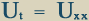— General solutions of PDE
— Particular and general representative solutions of PDE
— Boundary and initial conditions
— Well-posed and ill-posed problems
— Classification of second-order PDE
— Method of separation of variables
— Eigenvalues and eigenfunctions
— Method of characteristics
— Self-similar solutions
— Generalized functions. Dirac function
— Some properties of solutions of elliptic PDE
— Some properties of solutions of hyperbolic PDE
— Some properties of solutions of parabolic PDE
— Single-valued analytic functions
— Cauchy-Riemann equations
— Singularities and residues
— Method of sources and sinks
Introduction to fractal geometry.

##### ADVANCED ANALYSIS OF MECHANICAL SYSTEMS
— Some basic models of continuum media. Ideal fluid, perfect gas, elastic material. Ideal-plastic material, hardening-plastic material. Viscous fluids: Newtonian and non-Newtonian ones. Some rheological models. Laws of conservation. Derivation of ordinary and partial differential equations.
— Boundary layer approach as applied to the solution of ordinary and partial differential equations.
— Laplace's and Fourier's transforms as applied to solution of boundary value problems for ordinary and partial differential equations.
— Stationary and quasi-stationary dynamic systems on the phase plane. Stability and instability.
— Wave mechanics. Fourier series, particle-wave duality, spectral analysis, uncertainty principle of Heisenberg, causality principle.
— Numerical methods. Iteration method, fixed point theorem. Fredholm integral equations, ordinary and partial differential equations. Finite difference methods applied to Cauchy problem and some boundary value problems for ordinary and partial differential equations.
— Finite elements method.
— Variational methods.
— Linear finite difference equations.
— Multi-valued analytical functions. The principle of analytic continuation. Calculation of integrals in complex domain. Motion of cylinder in ideal fluid. The theory of wing. Conformal mapping.

###### MECHANICAL VIBRATIONS ANALYSIS
— The theory of harmonic oscillator. Free vibrations. Spring-mass model. Basic assumptions of the model. Governing equation. Solution and analysis. Total energy. The phase plane. The singular point. State of equilibrium and its stability.
— Some mechanical models of harmonic oscillator. Simple pendulum. Geometrical pendulum. Compound pendulum. Torsional pendulum. Beam pendulum. Oscillating circuit. Derivation of governing equation using Langranges's function. About 20 different types of harmonic oscillators given as homework problems.
— Complex vector of harmonic oscillator. Superposition of harmonic oscillations. Beats. Forced and excited vibrations. Resonance. Harmonic analysis of periodic functions.
— Harmonic oscillator with viscous friction. Damped vibrations. Direct investigation of the differential equation. Overdamped a periodic process. Oscillator with small mass.
— Non-linear spring in mass-spring model. Phase plane. Some examples.
— Linear oscillators with two degrees of freedom. Using Langrange's function. Some examples. Characteristic equation. Eigenfrequencies or natural frequencies. Principal coordinates.
— Linear oscillators with many degrees of freedom. Characteristic equation. Eigenfrequencies or natural frequencies. Resonance.
— Numerical analysis of eigenproblems. Basic characteristics of eigenproblems. The power method. The inverse power method. The direct method. Iterative and other methods.

— Review: one degree of freedom systems. Free, forced, damped and undamped vibrations. Forced support vibration, Newton's law in non-inertial coordinate frame. Effective stiffness calculation for combined bar-beam-string-plate systems.
— Non-linear conservative systems. Free and forced vibrations. Piecewise-linear systems. Numerical solution.
— Non-linear non-conservative systems. Self-excited vibrations. Van der Pol's equation. Kryloff- Bogoluboff method.
— Parametric resonance. Mathieu's equation. The Ince-Strutt diagram.
— Inelastic (especially, viscoelastic) material damping.
— Systems with multiple degrees of freedom. Some models. General analysis. Frequencies and mode shapes for undamped systems. Principal or normal coordinates. Damping in multidegree systems.
— Continuous systems with infinite number of degrees of freedom. Longitudinal vibrations of prismatic bars. Free and forced vibrations. Prismatic bar with a mass or spring at the end. The problem of bar impact.
— Torsional vibrations of shafts.
— Transverse vibrations of beams.
— Transverse vibrations of membranes and plates.
— 3D waves in continua.
— Stability analysis. Introduction to Liapunoffs method.

### CLASSICAL DYNAMICS

— Review. Vector algebra and curvilinear coordinates.
— Generalized coordinates. Generalized speeds. Configuration constraints. Motion constraints.
— The theory of gravitation. Conservative forces.
— Momentum conservation law. Motion of a variable mass. The theory of rocket flight.
— Velocity and acceleration in curvilinear coordinates.
— Differentiation in different reference frames. Inertial and non-inertial reference frames.
— Introduction to Lagrangian mechanics.
— Energy, work, power. Impact.
— Gyroscopes and tops. Eulerian angles.
— Euler's equations.
— Introduction to Hamiltonian mechanics.
— Introduction to relativistic mechanics.
— General principles, conservation laws, and some of their applications to fluid and solid mechanics.

#### SYNTHESIS OF ENGINEERING MECHANICS

— Concept of continuum in physics and engineering.
— ID mechanics of materials: constitutive equations.
— General incremental form of constitutive equations.
— Stress and strain tensors. Principal stresses. Maximum shear stress.
— Stress analysis. Theories of failure.
— Pressure cylinders and spinning disks. Stress concentration.
— Bending of circular plates.
— Elastic foundations.
— Membrane shells.
— Microcracking in composite materials.
— Rock cracking.

— Review of classic linear fracture mechanics. Stress intensity factor. Local fracture criterion and fracture toughness.
— Surface energy of solids in terms of invariant or path-independent integral.
— Adhesion energy of different solids in terms of invariant or path-independent integral. Analysis of basic problems: a thin film on a substrate, a thin plate on a substrate, two bonded membranes, two bonded beams, and a thin film deposited on a thin plate.
— Debonding of two different elastic-plastic and viscoelastic materials.
— Crack nucleation: impinging a dislocation upon an interface, encounter of two dislocation pileups, and hole coalescence in amorphous materials.
— Fracture or failure waves in brittle materials. Explosion as a process of liberation of internal energy. Energetic materials.
— Dislocation emission. Nanomechanics of dislocation generation and crack growth in polycrystalline materials. Screw shear model.
— Super-deep penetration at the Rayleigh speed. Rayleighon.
— Fractal cracks in solids. Fractal analysis of failure.

###### CONDUCTION HEAT TRANSFER
— Basic physical laws: Conduction (Fourier's law), convection (Newton's law) and radiation (Stefan- Boltzmann's law). Combined radiation/convection.
— General theory of heat conduction for 3D non-homogeneous, anisotropic and time-dependent media (unsteady process). Linear relations of irreversible thermodynamics. Onsager's rule. Law of energy conservation. Tensor and vector formulations of the general equation.
— Simplified theory for homogeneous, isotropic and time-independent media. Unsteady and steady-state processes. Boundary conditions. Cylindrical and spherical coordinates. Insulated duct of variable cross-section. Duct of variable cross-section with the lateral surface subject to convective heat exchange.
— One-dimensional steady-state heat conduction: the slab, the cylinder, the sphere. Composite medium. Thermal contact resistance. Critical thickness of insulation. Finned surfaces. Temperature-dependent conductivity. Lumped system analysis.
— Transient (unsteady) conduction: the slab, the cylinder, the sphere. Transient (unsteady) conduction in semi-infinite solids. Self-similar solutions. Product solutions.
— Basic dimensionless parameters/numbers of heat transfer: Biot, Fourier, Prandtl, Pecklet, Nusselt, Graetz, Eckert, Stanton, Grashof. Use of charts.
— Phase transition and Stefan's problems with unknown boundary. Problem of melting sphere and hail nucleation. Heat regimes of the Sun and Earth.
— Two-dimensional, steady-state heat conduction. Complex variables. Method of sources and sinks.
— Finite-difference methods for solving heat conduction boundary value problems.

## FATIGUE AND FAILURE ANALYSIS

— Kinetic theory of fracture.
— Theory of damage accumulation.
— Linear and non-linear damage.
— Fractography.
— ID stress. Failure. Fatigue. Creep.
— Plasticity. Bending of multi-layer beams and plates.
— Plastic deformation, brittle failure, buckling, fatigue, hydrogen embrittlement, corrosion, creep, erosion, wear, impact, thermal fatigue, stress corrosion, corrosion fatigue and other causes of failure.
— 2D and 3D stress. Yielding criteria. Fracture criteria.
— 3D stress-strain equations and models.
— Corroded zones, dislocations and cracks are the main physical elements of induced damage.
— Uniform corrosion. Rust. Oxide film growth.
— Hydrogen diffusion.
— Coatings and films.
— Electrochemical corrosion mechanism.
— Fatigue life estimates for structure components.
— Mixed mode cracking.
— Subcritical crack growth and life time prediction.
— Fatigue nanocracks, microcracks, and macrocracks in metals.
— Hydrogen-enhanced crack growth in metals.
— Stress corrosion.
— Fatigue and corrosion problems in composite materials.
— Humidity effects in metals, glass and rock.

### BOUNDARY VALUE PROBLEMS OF FUNCTION THEORY

— Mechanical interpretation of the theory of function of a complex variable. Liouville's theorem. Cauchy's theorem. Analytic continuation and Riemann surfaces.
— Cauchy integrals and their application to some problems of engineering and mathematical physics. Principal value of the contour Cauchy integral. Sokhotsky-Plemelj equation.
— Riemann boundary value problems and their application to engineering and mathematical physics. Gakhov's index. Carleman's method.
— Hilbert boundary value problems and their application in engineering and mathematical physics.
— Singular integral equations and their application in engineering and mathematical physics. Cauchy kernel and Hilbert kernel. Characteristic equation.
— Singular integral-differential equations and their application in engineering and mathematical physics. Using Chebyshev's polynomials.
— Wiener-Hopf and Noble-Jones methods of solving mixed boundary value problems.

— Physical foundation of the theory of elasticity. Hooke's law.
— Navier's and Cauchy's equations of the theory of elasticity.
— Boundary value problems for a boundless space and plane.
— Thermoelasticity. Bimetal problem. Thin films.
— Kolosov equations for plane stress and plane strain.
— First and second boundary value problems for a half-space and half-plane.
— Parabolic and hyperbolic bodies. Neuber's solutions.
— Plane with an elliptic hole. Muskhelishvili's method.
— Mixed problems. Galin's method.
— Hertz's problem. Slip and friction on a contact area.
— Contact adhesion and cohesion problems.
— Anisotropic materials. Lekhnitsky's method. Dynamic waves. Some models of earthquakes.
— Functional-invariant solutions by Smimoff-Soboleff.

##### BOUNDARY PROBLEMS IN ENGINEERING
— Derivation of ordinary and partial differential equations (ODE and PDE) and their reduction to first order systems of ODE and PDE.
— Diffusion - type problems.
— Hyperbolic - type problems.
— Elliptic - type problems.
— Analytical methods: using general solution and some specific properties of equations; separation of variables and choice of coordinates; integral transforms; using self-similarity and symmetry properties; perturbation method; boundary-layer method.
— Numerical methods: finite differences, Monte Carlo, finite elements. Variational methods.
— Introduction to fractal analysis. Some engineering applications.
— Introduction to the mathematical theory of catastrophe. Some engineering applications.

###### MECHANICS & MATERIALS SCIENCE
— Requisite mathematics. Equilibrium and forces (Archimedes). Geometry of space and time (Pyphagor, Euclid). Vector algebra and linear equations (Descartes). Calculus elements (Leibniz). Elements of ordinary differential equations (Euler).
— Pressure in fluids under static conditions (Archimedes law).
— Rod extension test (Leonardo da Vinci). Failure force (Galileo Galilei). Internal tension in solids (Leibniz and Jakob Bernoulli).

 Normal stress, strain and elasticity law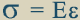, introduced by Euler and called Hooke's

law by him (constant  E  was called Young's modulus, who later measured the value of  E   for several materials).

— Statically-determinate structures and trusses. Spherical and cylindrical pressure vessels.

 — Shear stress and strain. Shear modulus in elasticity law,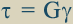(Coulomb).

— Poisson's effect. Poisson's ratio.

 — 3D stressesand 3D strains.

Equilibrium and motion equations (Cauchy). 3D Hooke's law (Cauchy, Navier, Green).
— 2D or plane strain and stress. Stresses and strains. Mohr's circle for plane strain. Stress transformation equations. Tractions.
— Material properties. Water, ice, rocks, wood, soils, metals, ceramics, and glass. Solid and liquid phase. Elastic properties. Polycrystalline and amorphous materials. Plasticity. Creep. Fatigue.

 — Torsion of solid or hollow circular shafts (Coulomb). Shear stress,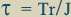,
 and twist, where T is the torque, r is the distance of a point from
 the center of symmetry, and  J  is the integral ofover section area (polar moment of

inertia).

 — Beam bending or flexure (Euler). Normal stress,, where M is the bending
 moment,  y  is the distance of a point from the neutral axis, and  I  is the integral of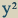over the section area (moment of inertia). Deflections.

 Equation of elastic line of a beam,, where  E  is Young's modulus,

and  u = u'(x)  is the deflection of the neutral line,

 so that u'(x) is the curvature of this line.

— Statically indeterminate beams and trusses.
— Combined extension, torsion, and flexure of circular shafts. Superposition principle (Euler).
— Columns. Buckling (Euler).
— Thermal stresses. The problem of bimetal beam (Timoshenko).

## NEW COURSES OFFERED BY DR. CHEREPANOV AND APPROVED BY THE CURRICULUM COMMITTEE

— Classic Topics of Non-Linear Mechanics (EGM 7 , for PhD students in fluid or solid mechanics).
— Field Theory (EML 5 , for MS students in fluid or solid mechanics or heat transfer).
— Mechanics of Vortex and Separated Flows (EML 7 , for PhD students in fluid mechanics).
— Advanced Modeling in Engineering (EML 6 , for PhD students in fluid or solid mechanics).
— Advanced Mechanics of Composite Materials (EMA 6 , for MS/PhD students in materials science).
— Mechanics of Fluid Flow in Porous Media (EML 6 , for MS/PhD students in fluid mechanics or heat transfer).

Syllabus of these courses follow below.

### CLASSIC TOPICS OF NON-LINEAR MECHANICS

— Non-linear dynamic systems. Singular points. Topology of trajectories on the phase plane. Limit cycles.
— Flows of ideal incompressible fluid with free stream lines. Cavities and caverns. Problems by Helmholtz, Rayleigh, Kirchhoff, Jukovsky, Villa, Chaplygin, Levi-Civita, Sedov, Taylor, and Lavrentiev.
— Perfectly plastic solids. Problems by Prandtl, Hill, and Sokolovsky.
— Elastic-plastic problems by Trefttz, Sokolovsky, Galin, McClintock, and Cherepanov.
— Contact problems for plates and membranes.

#### FIELD THEORY

— Review of basic equations of the theory of potential.
— Properties of harmonic functions. 3D and 2D problems.
— Conformal mapping.
— Potential flow of an ideal fluid.
— Harmonic elastic fields. Screw dislocations.
— Temperature field.
— Electrostatic and magnetostatic field.
— Diffusion and mass transport.
— Penetration mechanics.
— Lift of a wing.
— Impact of a body upon a fluid.
— Ocean waves.

##### MECHANICS OF FLUID FLOW IN POROUS MEDIA
— Physical properties of rocks and soils as porous materials.
— Water, air and oil as main physical fluids penetrating through porous materials.
— Darcy's law.
— General equations of fluid flow in porous media.
— The problems of ground waters. Depression line.
— Flows in seams and beds.
— Flow under a dam using complex variables.
— Flow in canals and rivers.
— The problem of contamination flow in thin holes and leaks in a porous medium.
— Problem of oil sources, wells and boreholes. Opening-up problems.
— Non-linear laws. Residual oil.
— Concept of double continuum. Biot's equations.
— Non-stationary flows of a fluid and gas.
— Theory of fluidization of packed beds. Fluidized beds.
— Biomechanical applications. Lungs.

— Introduction. Mathematical modeling as an heuristic discipline based on some principles.
— Black-box problem. Linear structure identification. Mach's principle.
— Singular problems. Matching asymptotes principle.
— Non-uniqueness problem. Selection principle.
— High-modulus rod in 3D space. Boundary layer principle.
— Global modeling using invariant integrals.
— System of many dislocations or vortices. Quasi-thermodynamics principles.
— Optimal design as an inverse problem of heuristic modeling. Equistrength principle.
— Fluidized bed incipiency. Local modeling principles.
— Scaling laws. Principles of fractal modeling.

## MECHANICS OF VORTEX AND SEPARATED FLOWS

— Vortex flow analysis. One vortex in ideal fluid space. Many bound and free vortices. Karman's vortex streets behind a cylinder. Vortex arrays and their stability. Separation lines as limits of vortex streets. Quasi-temperature of vortex arrays. Conservation laws.
— Some fluid models with discrete vortices.
— Dissipation of a vortex in viscous fluid.
— Vortex nucleation and shedding. Laminar boundary layer and separation criteria.
— Technique of numerical method of discrete vortices and some results of numerical experiments.
— Some classic separated flows. Calculation of drag force.
— Modeling of separated fluid flows. Some theorems.

### ADVANCED MECHANICS OF COMPOSITE MATERIALS

— Review of manufacturing technologies and basic properties of composite materials.
— Theory of reinforcement. Reinforcement of structure by stringers. Riveted panels.
— Reinforcement of matrix by fibers. Shear lag theory and its modification.
— Theory of pull-out and push-in.
— Thermal microstresses. Local breakage.
— Delamination theory. Protection films and coatings.
— Optimal design of composite materials.
— Micro-mechanisms and laws of damage of composite materials. Contact stress
concentration. Fatigue and corrosion.